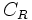# Elastic collision

(diff) ← Older revision | Latest revision (diff) | Newer revision → (diff)
Jump to: navigation, search

## Definition

A collision between two (or more) bodies is termed an elastic collision or perfectly elastic collision if the total kinetic energy of the bodies after the collision equals the total kinetic energy of the bodies before the collision. In other words, there is no conversion from kinetic energy to other forms of energy during the collision.

Elastic collisions are an ideal approximation that may not occur in practice, hence the term perfectly elastic is sometimes used to describe such collisions.

## Related notions

• Coefficient of restitution: Usually denoted$e$ or$C_R$, this number, which typically varies between$0$ and$1$, measures the bounciness of a collision. An elastic collision has coefficient of restitution equal to$1$, and a perfectly inelastic collision has a coefficient of restitution equal to$0$.
• Perfectly inelastic collision: A collision after which the two bodies stick to each other, i.e., their relative position remains the same as their relative position at the time of the collision.